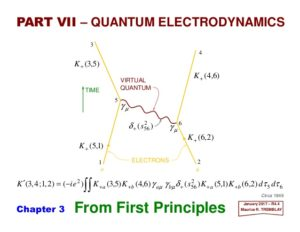# Incorporating gravity into Quantum electrodynamics or QED in terms of the field properties of space-time.

Einstein’s Explanation of the Unexplainable

Quantum electrodynamics or QED is the relativistic quantum field theory of electrodynamics. It’s a theoretical framework which combines classical field theory, special Relativity and the quantum mechanical properties of particles as excited states of quantum fields, which it assumes are more fundamental than the particles. However, it has not been able to derive gravity in terms of that field.

While in Relativity the exchange of energy between particles is derived terms of the evolution of its field properties caused by an electromagnetic wave and derives gravity in terms of an increase in its local energy density.

However, QED’s explanation of the double slit experiment also suggests a wave is responsible of the evolution of particles.“This is because it assumes when an electron passes through the double slit, the wave properties it associates with its quantum field interfere. It says this interference results in the wave collapsing to form a particle and its position is defined at the location where the electron quantum field is elevated. So, an electron or particle cannot appear in any locations where the electron quantum field interferes destructively. This gives rise to the interference pattern on the back screen.”

However, as was mentioned earlier Einstein derived gravity in terms of increase in the local energy density of a space-time field.

This means one MAY be able to integrate gravity into QED by showing a how the interaction of wave in both a Relativistic and QED field is responsible for creating a quantized increase the local energy density their respective fields.

This can be done by using the science of wave mechanics and the fact Relatively tells us wave energy would move continuously through a space-time field unless it is prevented from doing so by someone observing or something interacting with it. This would result in its energy being confined to a three-dimensional field consisting of only space. The science of wave mechanics also tells us the three-dimensional “walls” of this confinement will result in its energy being reflected back on itself thereby creating a resonant or standing wave in three-dimensional space. This would cause the wave energy to collapsed and be CONCENTRATED at the point in space resulting in increasing the energy density of the field at that point. Additionally, wave mechanics also tells us the energy of a resonant system, such as a standing wave which this confinement would create can only take on the discrete or quantized values associated with its fundamental or a harmonic of its fundamental frequency.

This mechanism is similar to how QED defines the collapse the field properties of a particle as being caused interference of its field properties.

This means both QED and Relativity can define the quantization of gravity in terms of the collapse of the wave properties of their respect fields. QED assumes the wave properties it associates with a quantum field interfere and causes it to COLLASPE into a particle thereby increasing the energy density in that field. While as was shown above the science of wave mechanics also tells us if a wave a space-time field is prevented from moving through it would cause it to COLLASPE resulting in a quantized increase in energy density of the three-dimensional field it occupies.

Therefore, they both can define why gravity is quantized if one assumes as Einstein did it is a result of terms of an increase in in the local energy density of space.

However, because we cannot observe a quantum field the assumption made by QED that its interference with itself results in it becoming quantized is NOT repeat NOT supported by observations. Yet, we can observe how waves interfere with the three-dimensional aspects of Einstein four-dimensional space-time field to from quantized resonant systems as was show above to allow one to define quantum gravity.

What we have to decide as Scientists, should we use observable properties of Einstein space-time field to construct and test hypotheses and theories on the quantization of gravity or should we base them on the unobservable properties of a QED field.